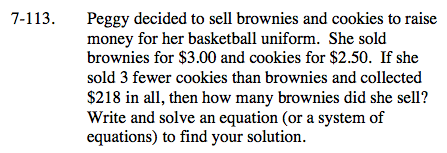Home > CAAC > Chapter 7 > Lesson 7.3.4 > Problem7-113

7-113.Peggy decided to sell brownies and cookies to raise money for her basketball uniform. She sold brownies for $3.00 and cookies for$2.50. If she sold 3 fewer cookies than brownies and collected \$218 in all, then how many brownies did she sell? Write and solve an equation (or a system of equations) to find your solution. Homework Help ✎Write an equation for the problem.

x − 3 = y
3x + 2.5y = 218

x = the number of brownies
y = the number of cookies.

Substitute for y in the second equation and solve for x, the number of brownies.

3x + 2.5(x − 3) = 218
3x + 2.5x − 7.5 = 218
5.5x = 225.5
x = 41